# Samacheer Kalvi 6th Maths Solutions Term 1 Chapter 4 Geometry Ex 4.3## Tamilnadu Samacheer Kalvi 6th Maths Solutions Term 1 Chapter 4 Geometry Ex 4.3

Question 1.
Observe the diagram and fill the blanks.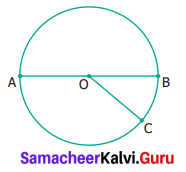(i) ‘A’, ‘O’ and ‘B’ are ______ points
(ii) ‘A’, ‘O’ and ‘C’ are ______ points
(iii) ‘A’ ‘B’ and ‘C’ are _____ points
(iv) ______ is the point of concurrency
Solution:
(i) collinear points
Hint: Points on a line.
(ii) non collinear points
Hint: Points not on a line
(iii) end points/non collinear points
(iv) O is the point of concurrency.
Hint: A points where lines meet

Question 2.
Draw any line and mark any 3 points that are collinear.
Solution: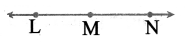L, M, N are collinear points

Question 3.
Draw any line and mark any 4 points that are not collinear.
Solution: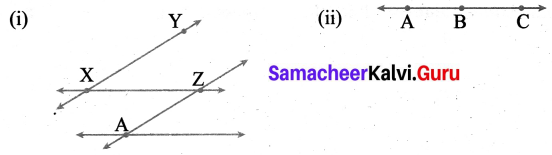X, Y, Z and A are non-collinear points.

Question 4.
Draw any 3 lines to have a point of concurrency.
Solution: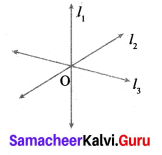l1, l2 and l3 are the concurrent lines.
‘O’ is the point of concurrency.Question 5.
Draw any 3 lines that are not concurrent. Find the number of points of intersection.
Solution: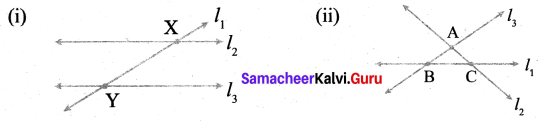l1, l2 and l3 are non concurrent lines.
A, B and C are the 3 points of intersection.
There are two points of intersection X and Y

Objective Type Questions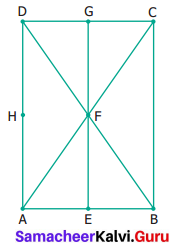Question 6.
A set of collinear points in the figure are ……….
(a) A. B, C
(b) A, F, C
(c) B, C, D
(d)A,C,D
Solution:
(b) A, F, C

Question 7.
A set of non-collinear points in the figure are _____
(a) A, F, C
(b) B, F, D
(c) E, F, G
(d) A, D, C
Solution:
(d) A, D, C
Hint: Non-collinear points are points which are not on a line.

Question 8.
A point of concurrency in the figure is ……..
(a) E
(b) F
(c) G
(d) H
Solution:
(b) F